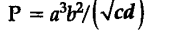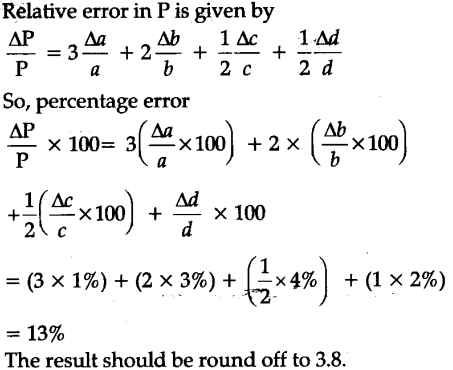# A physical quantity P is related to four observables a, b, c and d as follows:

A physical quantity P is related to four observables a, b, c and d as follows:The percentage errors of measurement in a,b,c and d are 1%, 3%, 4% and 2% respectively.What is the percentage error in the’quantity P ? If the value of P is calculated using the above -.relation turns put to be 3.763,to what value should you round off the result ?